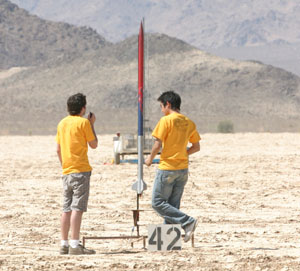# E80 Spring 2016

## Temperature Measurements### Reference Materials

The PDF of the 2016 lecture is here. The .pptx file of the 2016 lecture notes is here. The streaming video of the lecture may eventually be here. In the meantime, log on to Sakai, go to the HM ENGR80.X 2016 tab (where X is your section number, 1,2,3, or 4), click on the MediaSite link in the left navigation bar, if necessary click on the press here to launch link, and then watch the E80 - 2016S - Rm. Recital Hall_2/4/2016 video.

The PDF of the 2011 lecture is here.

The PowerPoint of the 2009 Lecture is found here.

The LabVIEW manual page for General Linear Least-Squares Fit is found here. MATLAB's instructions for General Linear Least-Squares Fit are found here. An example m-file (which will work in LabVIEW MathScript or MATLAB) which performs linear regression is found here. An example m-file (which will work in LabVIEW MathScript or MATLAB) which performs regression for the Steinhart-Hart constants is found here.

The PowerPoint of the 2008 lecture is found here.